Courses

# Test: Entropy - 3

## 15 Questions MCQ Test Topicwise Question Bank for Mechanical Engineering | Test: Entropy - 3

Description
This mock test of Test: Entropy - 3 for Mechanical Engineering helps you for every Mechanical Engineering entrance exam. This contains 15 Multiple Choice Questions for Mechanical Engineering Test: Entropy - 3 (mcq) to study with solutions a complete question bank. The solved questions answers in this Test: Entropy - 3 quiz give you a good mix of easy questions and tough questions. Mechanical Engineering students definitely take this Test: Entropy - 3 exercise for a better result in the exam. You can find other Test: Entropy - 3 extra questions, long questions & short questions for Mechanical Engineering on EduRev as well by searching above.
QUESTION: 1

Solution:
QUESTION: 2

Solution:
QUESTION: 3

### Clausius inequality is stated as

Solution:

If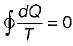Cycle is reversible
If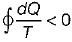Cycle is irreversible
IfCycle is impossible
Hence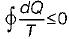For cycle is possible
This is known as Clausius inequality.

QUESTION: 4

When a system undergoes a process such that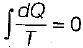and ΔS > 0, the process is

Solution:
QUESTION: 5

A certain amount of fluid at temperature T1 is mixed with an equal amount of the same fluid at temperature T2 in an insulated container with total fluid as the system, consider the following statements
I. Energy of the system is conserved
II. Entropy of the system is conserved
III. Entropy of the system increases
IV. Entropy of the system decreases
Q. Which of the above statements is/are true?

Solution:
QUESTION: 6

Which one of the following statements applicable to a perfect gas will also be true for an irreversible process

Solution:

δQ = dU+ PdV... Applicable for a closed system when only PdV work is present. This is true only for a reversible process.
δQ = TdS ...Applicable for a reversible process.
TdS = dU + PdV... Applicable for any process reversible or irreversible, undergone by a closed system, since it is a relation among properties which are independent of the path.

QUESTION: 7

The entropy change for any closed system which undergoes an irreversible adiabatic process

Solution:
QUESTION: 8

Consider two subsystem 1 and 2 containing same fluid and having same mass m; but at Temperature T1 and T2(T> T2) enclosed in an adiabatic enclosure separate by a partition, if the partition is removed and the fluids are allowed to mix. The entropy change of process is

Solution:

Subsystem 1 having a fluid of mass m1 specific heat c1 and temperature t1 and subsystem 2 consisting of a fluid of mass m2, specific heat c2, and temperature t2, comprise a composite system in an adiabatic enclosure figure. When the partition is removed, the two fluids mix together, and at equilibrium let tf be the final temperature, and t2 < tf < t1 Since energy interaction is exclusively confined to the two fluids, the system being isolated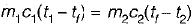∴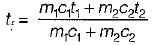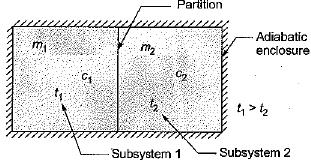Entropy change for the fluid in subsystem1,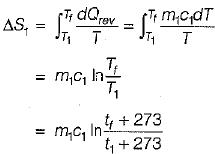This will be negative, since T> Tf
Entropy change for the fluid in subsystem 2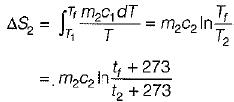This will be positive, since T2 < Tf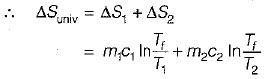ΔSuniv will be positive definite, and the mixing process is irreversible.
Although the mixing process is irreversible, to evaluate the entropy change for the subsystem, the irreversible path was replaced by a reversible path on which the integration was performed.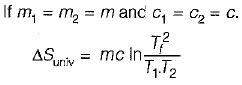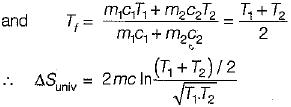QUESTION: 9

For the isentropic expansion of an ideal gas from the initial conditions P1,Tto the final conditions P2,T2, which one of the following relations is valid?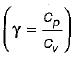Solution:

For isentropic expansion, PVγ = constant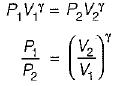from ideal gas law,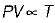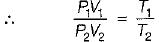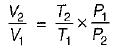Also,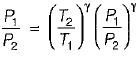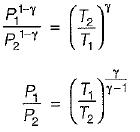QUESTION: 10

The change in entropy of the system, ΔSsys, undergoing a cyclic irreversible process is

Solution:

Every process proceeds in such direction that total entropy change associated with it will be positive.

QUESTION: 11

In a reversible isothermal process, an ideal gas expands to four times its initial volume. The change in entropy is

Solution:
QUESTION: 12

High pressure steam is expanded adiabatically and reversible through a well insulated turbine which produces some shaft work. If the enthalpy change and entropy change across the turbine are represented by ΔH and ΔS, respectively, for this process:

Solution:

For reversible adiabatic process, ΔS = 0 but ΔH ≠ 0.

QUESTION: 13

A system undergo a state change from 1 to 2, according to second law of thermodynamics for the process to be feasible, the entropy change (S- S1) of the system

Solution:
QUESTION: 14

The following four figure have been drawn to represent a fictitious thermodynamic cycle, on P - V and T - S planes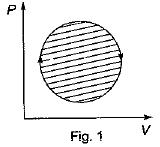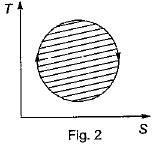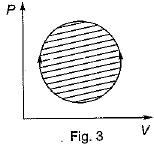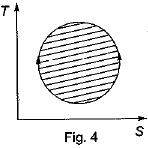According to first Saw of thermodynamics, equal areas are enclosed by

Solution:

We know that:
For closed cycle change in internal energy is zero First law of thermodynamic for closed system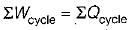Hence, equal area are enclosed by figures 1 and 2.

QUESTION: 15

Four process of thermodynamic cycle are shown in figure on P-V diagram in the sequence 1 -2-3-4. The corresponding correct sequence of these process in the T-S plane shown in figure will be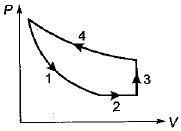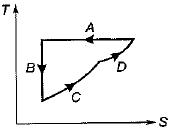Solution: